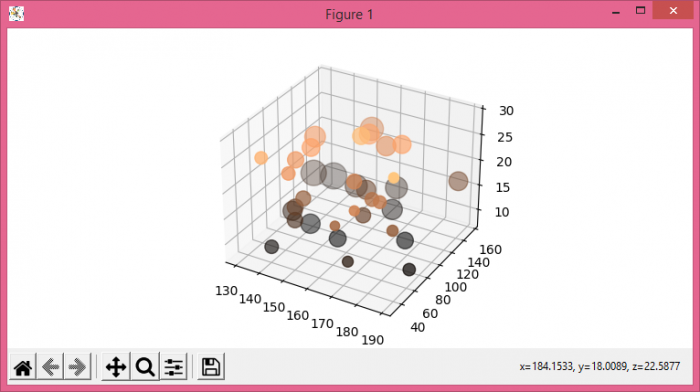# Plot scatter points on a 3D projection with varying marker size in Matplotlib

MatplotlibPythonData Visualization

To plot scatter points on a 3D projection with varying marker size, we can take the following steps

• Set the figure size and adjust the padding between and around the subplots.
• Create xs, ys and zs data points using numpy
• Initialize a variable 's' for varying size of marker.
• Create a figure or activate an existing figure using figure() method.
• Add an axes to the current figure as a subplot arrangement using subplots() method.
• Plot the xs, ys, and zs data points using scatter() method.
• To display the figure, use show() method.

## Example

import numpy as np
from matplotlib import pyplot as plt
plt.rcParams["figure.figsize"] = [7.50, 3.50]
plt.rcParams["figure.autolayout"] = True
xs = np.random.randint(low=8, high=30, size=35)
ys = np.random.randint(130, 195, 35)
zs = np.random.randint(30, 160, 35)
s = zs / ((ys * 0.01) ** 2)
fig = plt.figure()
plt.show()